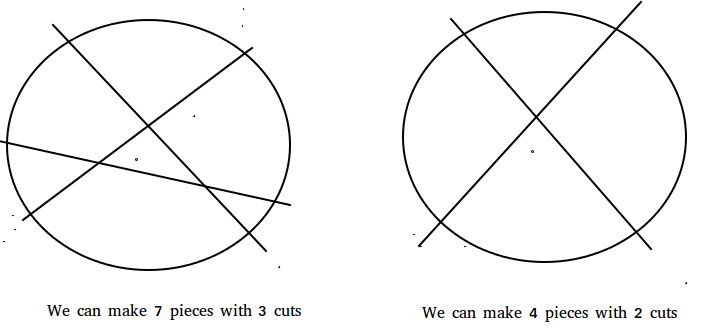The Lazy Caterer’s Problem

• Difficulty Level : Easy
• Last Updated : 30 Mar, 2021

Given an integer n, denoting the number of cuts that can be made on a pancake, find the maximum number of pieces that can be formed by making n cuts.
Examples :

Input :  n = 1
Output : 2
With 1 cut we can divide the pancake in 2 pieces

Input :  2
Output : 4
With 2 cuts we can divide the pancake in 4 pieces

Input : 3
Output : 7
We can divide the pancake in 7 parts with 3 cuts

Input : 50
Output : 1276Recommended: Please solve it on “PRACTICE” first, before moving on to the solution.

Let f(n) denote the maximum number of pieces
that can be obtained by making n cuts.
Trivially,
f(0) = 1
As there'd be only 1 piece without any cut.

Similarly,
f(1) = 2

Proceeding in similar fashion we can deduce
the recursive nature of the function.
The function can be represented recursively as :
f(n) = n + f(n-1)

Hence a simple solution based on the above
formula can run in O(n).

We can optimize above formula.

We now know ,
f(n) = n + f(n-1)

Expanding f(n-1) and so on we have ,
f(n) = n + n-1 + n-2 + ...... + 1 + f(0)

which gives,
f(n) = (n*(n+1))/2 + 1

Hence with this optimization, we can answer all the queries in O(1).
Below is the implementation of above idea :

C++

 // A C++ program to find the solution to // The Lazy Caterer's Problem #include using namespace std;   // This function receives an integer n // and returns the maximum number of // pieces that can be made form pancake // using n cuts int findPieces(int n) {     // Use the formula     return (n * ( n + 1)) / 2 + 1; }   // Driver Code int main() {     cout << findPieces(1) << endl;     cout << findPieces(2) << endl;     cout << findPieces(3) << endl;     cout << findPieces(50) << endl;     return 0; }

Java

 // Java program to find the solution to // The Lazy Caterer's Problem import java.io.*;   class GFG {     // This function returns the maximum     // number of pieces that can be made     //  form pancake using n cuts     static int findPieces(int n)     {         // Use the formula         return (n * (n + 1)) / 2 + 1;     }           // Driver program to test above function     public static void main (String[] args)     {         System.out.println(findPieces(1));         System.out.println(findPieces(2));         System.out.println(findPieces(3));         System.out.println(findPieces(50));     } }   // This code is contributed by Pramod Kumar

Python3

 # A Python 3 program to # find the solution to # The Lazy Caterer's Problem   # This function receives an # integer n and returns the # maximum number of pieces # that can be made form # pancake using n cuts def findPieces( n ):       # Use the formula     return (n * ( n + 1)) // 2 + 1   # Driver Code print(findPieces(1)) print(findPieces(2)) print(findPieces(3)) print(findPieces(50))   # This code is contributed # by ihritik

C#

 // C# program to find the solution // to The Lazy Caterer's Problem using System;   class GFG {     // This function returns the maximum     // number of pieces that can be made     // form pancake using n cuts     static int findPieces(int n)     {         // Use the formula         return (n * (n + 1)) / 2 + 1;     }           // Driver code     public static void Main ()     {         Console.WriteLine(findPieces(1));         Console.WriteLine(findPieces(2));         Console.WriteLine(findPieces(3));         Console.Write(findPieces(50));     } }   // This code is contributed by Nitin Mittal.



Javascript



Output :

2
4
7
1276

References : oeis.org
This article is contributed by Ashutosh Kumar .If you like GeeksforGeeks and would like to contribute, you can also write an article using write.geeksforgeeks.org or mail your article to review-team@geeksforgeeks.org. See your article appearing on the GeeksforGeeks main page and help other Geeks.